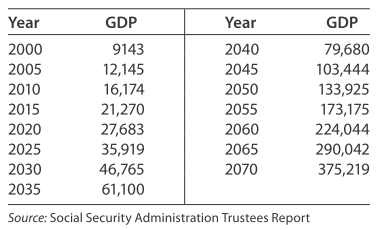# Modeling Gross domestic product The table shows U.S. gross domestic product (GDP) in billions of dollars for selected years from 2000 to 2070 (actual and projected). Model these data with a cubic function g = g ( t ) , where g is in billions of dollars and t represents the number of years past 2000. Report the model with three significant digit coefficients. Use the reported model to find the predicted instantaneous rate of change of the GDP in 2025. Interpret your answer to part (b).### Mathematical Applications for the ...

11th Edition
Ronald J. Harshbarger + 1 other
Publisher: Cengage Learning
ISBN: 9781305108042

#### Solutions

Chapter
Section### Mathematical Applications for the ...

11th Edition
Ronald J. Harshbarger + 1 other
Publisher: Cengage Learning
ISBN: 9781305108042
Chapter 9.4, Problem 62E
Textbook Problem
1 views

## Modeling Gross domestic product The table shows U.S. gross domestic product (GDP) in billions of dollars for selected years from 2000 to 2070 (actual and projected).Model these data with a cubic function g = g ( t ) , where g is in billions of dollars and t represents the number of years past 2000. Report the model with three significant digit coefficients. Use the reported model to find the predicted instantaneous rate of change of the GDP in 2025. Interpret your answer to part (b).

This textbook solution is under construction.

### Still sussing out bartleby?

Check out a sample textbook solution.

See a sample solution

#### The Solution to Your Study Problems

Bartleby provides explanations to thousands of textbook problems written by our experts, many with advanced degrees!

Get Started